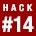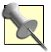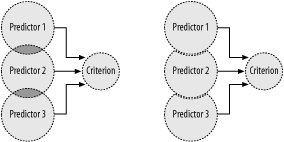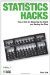# Hack 14. Use More Than One Variable to Predict AnotherThe super powers of predicting the future and seeing the invisible are available to any statistics hackers who feel they are worthy. Statisticians often answer questions and use correlational information to solve problems by using one variable to predict another. For more accurate predictions, though, several predictor variables can be combined in a single regression equation by using the methods of multiple regression.

"Graph Relationships" [Hack #12] discusses the useful prophetic qualities of a regression line. Those procedures allow administrators and statistical researchers to predict performance on assessments never taken, understand variables, and build theories about relationships among those variables. They accomplish these tricks using just a single predictor variable.

"Use One Variable to Predict Another" [Hack #13] presents the problem colleges have when deciding which applicants to admit. They want to admit students who will succeed, so they try to predict future performance. The solution in that hack uses one variable (a standardized test score) to estimate performance on a future variable (college grades).

Often, real-life researchers want to make use of the information found in a bunch of variables, not just one variable, to make predictions or estimate scores. When they want greater accuracy, scientists attempt to find several variables that all appear to be related to the criterion variable of interest (the variable you are trying to predict). They use all this information to produce a multiple regression equation.

#### Choosing Predictor Variables

You probably should read or reread "Use One Variable to Predict Another" [Hack #13] before going further with this hack, just to review the problem at hand and how regression solves it. Here is the equation we built in that hack for using a single predictor, ACT scores, to estimate future college admission:

Predicted GPA = -.24 + (ACT Scorex.16)

This single predictor produced a regression equation with output that correlated .55 with the criterion. Pretty good, and pretty accurate, but it could be better.

Imagine our administrator decides she's unhappy with the level of precision she could get using the regression line or equation she had built, and wants to do a better job. She could get a more accurate result if she could find more variables that correlate with college grades. Let's imagine that our amateur statistician found two other predictor variables that correlated with college performance:

• An attitude measure

• The quality of a written essay

Perhaps performance on a college attitude survey is collected by the college (scores range between 20 and 100), and is found to have some correlation with future GPA. Additionally, a score of 1 to 5 on a personal essay could correlate with college GPA and might be included in the multiple regression equation.

#### Building a Multiple Regression Equation

Let's look first at the abstract format of the regression equation in general. Then, we'll apply the tool to the task at hand. Here is the basic regression equation using just one predictor variable:

Criterion = Constant + (PredictorxWeight)

If you want to use more information, you can extend this equation to include more predictors. Here's an equation with three predictors, but you could expand the equation form to include any number of predictors:

Criterion = Constant +
(Predictor 1xWeight 1) +
(Predictor 2xWeight 2) +
(Predictor 3xWeight 3)

Each predictor has its own associated weight, which is determined through statistical formulas that are based on the correlation between the predictor and the criterion variable. The equations for this process are somewhat complex, so I won't show them here. (You're welcome.) In real-life regression equation building, computers are almost always used to produce multiple regression equations.I used the statistical software SPSS for many of the computations in this book, using data, often fictional, that I entered into SPSS data files. Microsoft's Excel is another handy tool for performing simple statistical analyses.

Using realistic data that we might find with three predictors that correlate with the criterion, as well as correlate with each other somewhat, we might produce a regression equation with values like this:

Predicted GPA = 3.01 +
(ACT Scorex.02) +
(Attitude Scorex.007) +
(Essay Scorex.025)

With the imaginary data I used on my computer to produce these weights, the overall equation predicted college GPA very well, finding a correlation of .80 between observed GPA values and predicted GPA values. This is much better than the .55 correlation of our single predictor.When we add two other predictors to the model (a description of a group of variables and how they are related), specifically the attitude measure and the essay score, the weight for the ACT score changes. This is because of the use of partial correlations instead of one-to-one correlations for each predictor. In addition, the constant changes. This is discussed later, in the "Why It Works" section of this hack.

#### Making Predictions and Understanding Relationships

To estimate what a prospective student's college performance will be, our administrator takes the scores for that student on each of the predictors and enters them into the equation. She multiplies each predictor score by its weight and adds the constant. The resulting value is the best guess for future performance. It might not be exactly right, of course (and, in fact, is most likely not exactly right), but it is a better guess than having no information at all.If you have no information at all and have to guess how a student will do in college, you should guess that she will earn the mean GPA, whatever that is for your institution.

What if you want to do more than just predict the future, and want to really understand the relationships between your predictors and the criterion? You might do this because you want to build a more efficient formula that doesn't require a bunch of information that isn't very useful. You also might do it just because you want to build theory and understand the world, you crazy scientist, you! The problem is that it is hard to know the independent contribution of each predictor by just looking at the weights.

The weights for each variable in a multiple regression equation are scaled to the actual range of scores on each variable. This makes it hard to compare each predictor to figure out which provides the most information in predicting the criterion. Comparing these raw weights can be misleading, as a variable might have a smaller weight just because it is on a bigger scale.

Compare the weight for ACT score with the weight for attitude score, for example. The weight of .02 for ACT is larger than the weight of .007 for attitude, but don't be fooled into thinking that ACT scores play a larger role in predicting GPA than attitude. Remember, GPA scores range from about 1.0 to 4.0, whereas attitude scores range from 20 to 100. A smaller weight for attitude actually results in a bigger jump on the criterion than does the larger weight for ACT scores.

Computer program results for multiple regression analyses often provide information in the format shown in Table 2-4.

##### Table Multiple regression results
CriterionNonstandardized weightsStandardized weights
Constant3.01-----
ACT scores.02.321
Attitude scores.007.603
Essay scores.025.156

The third column in Table 2-4 is more useful than the "Nonstandardized weights" values in identifying the key predictors and comparing the unique contributions of each predictor to estimating the criterion.Standardized weights are the weights you would get if you first convert all the raw data into z scores [Hack #26]: the distance of each raw score from the mean expressed in standard deviations.

The standardized weights have placed all predictors on the same scale. By doing this, the relative overlap of each predictor with the criterion can be fairly compared and understood. For example, with this data, it is probably appropriate to say that attitude explains twice as much about college GPA than does ACT performance, because the standardized weight for attitude is .603, about twice as much as the standardized weight for ACT scores (.321).

#### Why It Works

Multiple linear regression does a better job in predicting outcomes than simple linear regression because multiple regression uses an additional bit of information to compute the exact weights for each predictor. Multiple regression knows the correlation of each predictor with the other predictors and uses that to create more accurate weights.

This bit of complexity is necessary because if the predictors are related to each other, they share some information. They aren't really independent sources of prediction if they correlate with each other. To make the regression equation as accurate as possible, statistical procedures remove the shared information from each predictor in the equation. This produces independent predictors that come at the criterion from different angles, producing the best prediction possible.Imagine two predictor variables that correlate perfectly with each otherthat is, correlation equals 1.00. Using both variables in a regression equation would be no more accurate than using just one (doesn't matter which one) by itself. By extension, any overlap between predictors (i.e., any correlation between predictors greater or less than 0.00) is redundant information.

Figure 2-3 illustrates the use of multiple sources of independent information to estimate a criterion score.

##### Figure 2-3. Multiple predictors in multiple regressionThe correlation information used to determine the weight for each predictor in multiple regression is not the one-to-one correlation between a predictor and the criterion. Instead, it is the correlation between a predictor and the criterion when the overlap among all the predictors has been removed.

This process produces predictor variables that are somewhat different than the actual measure variables. By statistically removing (or controlling for) the shared information among predictors, the predictors are conceptually different than they were before. As Figure 2-3 shows, now they are independent predictors with a different "shape." The correlations between these altered variables and the criterion variable are used to produce the weights.Correlations between predictor variables and a criterion variable when all the redundant shared information has been statistically removed from the predictors are called partial correlations. Partial correlations are the one-on-one correlations you would get between each predictor and the criterion if the predictor variables do not correlate with each other.

#### Where Else It Works

Multiple regression is used every day by real people in the real world for one of two reasons. First, multiple regression allows for the construction of a prediction equation, so people can use scores on a group of variables that they have in front of them to estimate a score on another variable that they cannot have in front of them (because it is either in the future or cannot be measured easily for some reason). This is how the tool of multiple regression is used to solve problems in the world of applied science.

Multiple regression also allows for examination of the independent contribution that a group of variables make to some other variable. It allows us to see where there is information overlap among variables and build theories to understand or explain that overlap. This is how the tool of multiple regression is used to solve problems in the world of basic science.Statistics Hacks: Tips & Tools for Measuring the World and Beating the Odds
ISBN: 0596101643
EAN: 2147483647
Year: 2004
Pages: 114
Authors: Bruce Frey

Similar book on Amazon